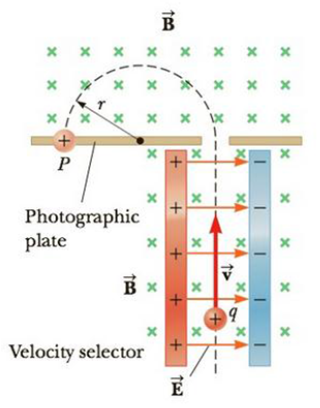Chapter 19, Problem 15P

Chapter
Section
Textbook Problem

Consider the mass spectrometer shown schematically in Figure P19.15. The electric field between the plates of the velocity selector is 9.50 × 102 V/m, and the magnetic fields in both the velocity selector and the deflection chamber have magnitudes of 0.930 T. Calculate the radius of the path in the system for a singly charged ion with mass m = 2.18 × 10−26 kg. Hint: See Problem 14.Figure P19.15 (Problem 15 and 21) A mass spectrometer. Charged particles are first sent through a velocity selector. They then enter a region where a magnetic field B → (directed inward) causes positive ions to move in a semicircular path and strike d photographic film at P.

To determine
The radius of the path in the system for a singly charged ion.

Explanation

Given info: The electric field between the plates of the velocity selector is 9.50×102Vm-1 . The magnetic fields in both the velocity selector and the deflection chamber are 0.930T . The ion is singly charged. The mass of the ion is m=2.18×1026kg .

Explanation:

From the velocity selector particle only with velocity v=EB will emerge out. Hence the velocity of the ions is,

v=EB       (1)

• E is the electric field
• B is the magnetic field

In the deflection chamber the centripetal force is supplied by the magnetic field.

Hence,

qvB=mv2r

• q is the charge of the ion
• m is the mass of the ion
• r is the radius of the circular path of the ion

Re-arranging for the radius,

r=mvqB       (2)

Combining (1) and (2),

r=mEqB2

Substitute 2.18×1026kg for m , 9

Still sussing out bartleby?

Check out a sample textbook solution.

See a sample solution

The Solution to Your Study Problems

Bartleby provides explanations to thousands of textbook problems written by our experts, many with advanced degrees!

Get Started